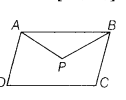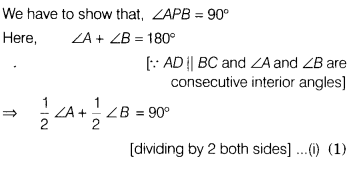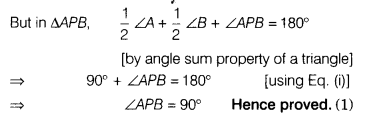# In a parallelogram, show that the angle bisectors of two adjacent angles intersect at right angles

In a parallelogram, show that the angle bisectors of two adjacent angles intersect at right angles.

Given, ABCD is a parallelogram such that angle bisectors of adjacent angles A and 6 intersect at point P